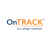4

# OnTRACK Grade 8 Math: Number and Operations

• Subject(s): Math
• Release date: 08-22-2015
• Tags: reasonableness, reasonable solutions, problem solving, square roots, irrational numbers, approximation, scientific notation, power of 10, order of magnitude, exponent, coefficient, project share, percents, order, OnTrack, integers, Grade 8 Math, fractions, decimal

## Description

Students will learn how to apply mathematical process standards to represent and use real numbers in a variety of forms.

## Standards

M.8.1.B, M.8.8.C, M.8.2.B, M.8.2.C, M.8.2.D

## Resources